# Bolted splice connection

$$Subcode: LRFDType of connection: Bolted splice connectionUnit system: MetricDesigned acc. to: AISC 360-16Investigated: Bolts in shear and bearingPlate material: A709, Gr. 50Bolts: 5/8'' A307Geometry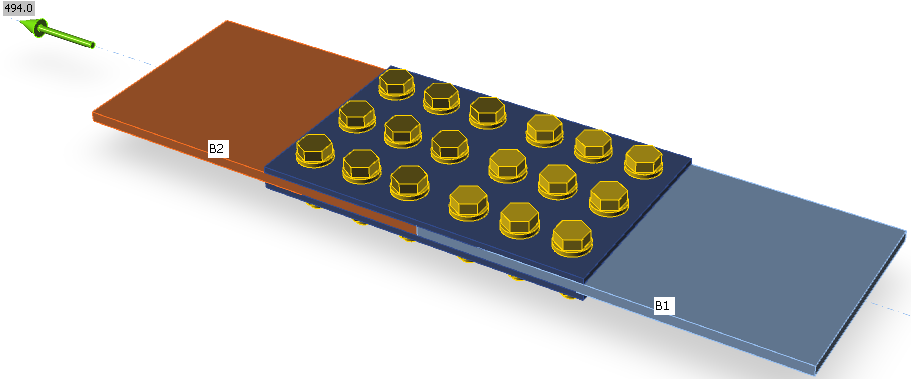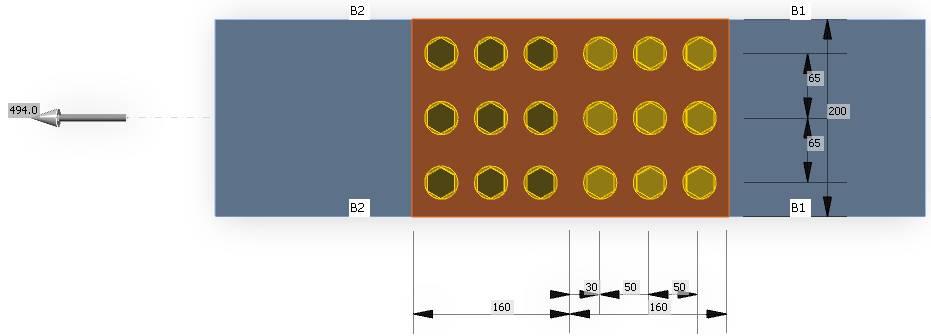Applied loadBolted splice connection is loaded by tension for the bolts in shear to be utilized at 100 %.ProcedureConcentrically loaded splice bolted connection is designed so that bolts fail in shear (AISC 360-16 – J3.6). Bolt strength in bearing (AISC 360-16 – J3.10) and strength of plates in tension (AISC 360-16 – J4.1) are also checked. The bolt threads are intercepted by the shear plane.Manual calculationBolt strength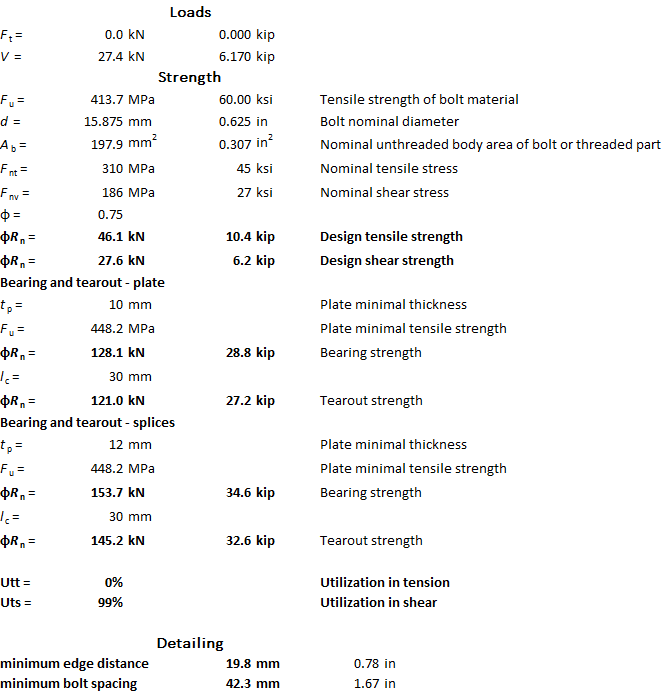The resistance of one shear plane of one bolt in shear is 27.6 kN. Each bolt has two shear planes and there are 9 bolts at each half of the splice. The resistance is therefore 2 ∙ 9 ∙ 27.6 = 496.7 kN.The bearing strength and tearout strength resist the force acting on both shear planes, i.e. 2 ∙ 27.6 = 55.2 kN. The smallest strength is the tearout strength of bolts near the edge of connected plates with the thickness of 10 mm, 121 kN. Other bolts have bearing strength of 128.1 kN and the decisive is the plate with the thickness of 10 mm. The sum of thicknesses of splices resisting in bearing is 12 mm.Strength of plates in tension$\phi R_n = \phi F_y A_g = 0.9 \cdot 344.7 \cdot 2000 = 620.46 \, \textrm{kN}$$\phi R_n = \phi F_u A_e = 0.75 \cdot 448.2 \cdot 1476 = 496.16\,\textrm{kN}$Check in IDEA StatiCa Connection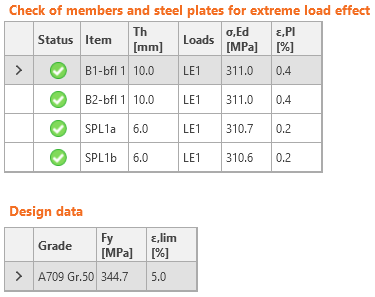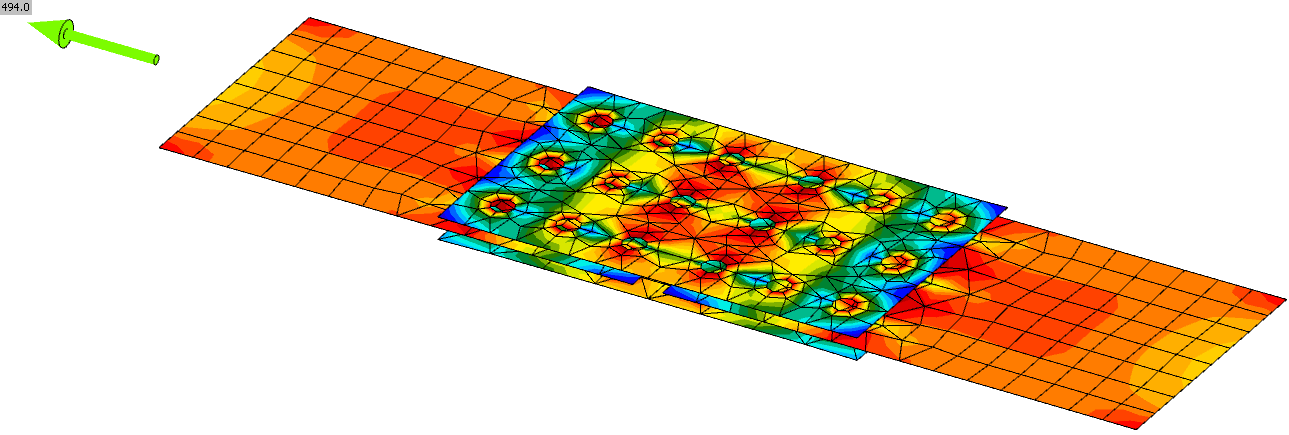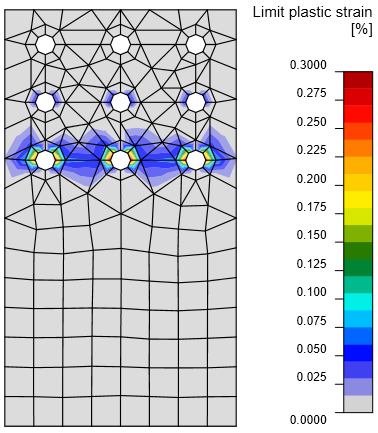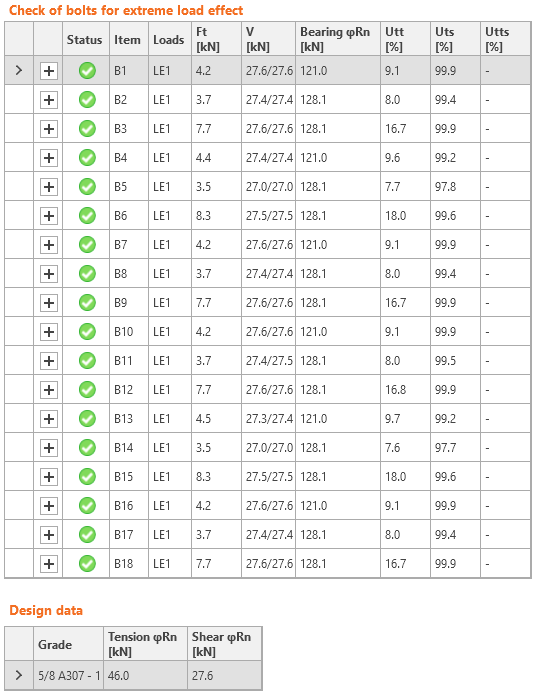There are some tensile forces in bolts due to plate deformation. Bolts are utilized at 99.9 % and plates are yielding. From the plastic strain at the connected plate, it can be seen that the reserve is not very high.ComparisonThe tensile forces in bolts are neglected in manual calculation but they do not affect the shear strength of bolts by much. The load resistance in IDEA StatiCa Connection is 494 kN while in manual calculation, it is 496 kN. In IDEA StatiCa Connection, bolts are decisive and the plates are yielding. In manual calculation, the bolts and plate tensile rupture have the same load resistance. The difference between results provided by software and manual calculation is only 0.4 %. Vzorové projekty Otevřít v aplikaci Viewer Stáhnout$$

## Vyzkoušejte program IDEA StatiCa

Stáhněte si zdarma zkušební verzi na 14 dní.